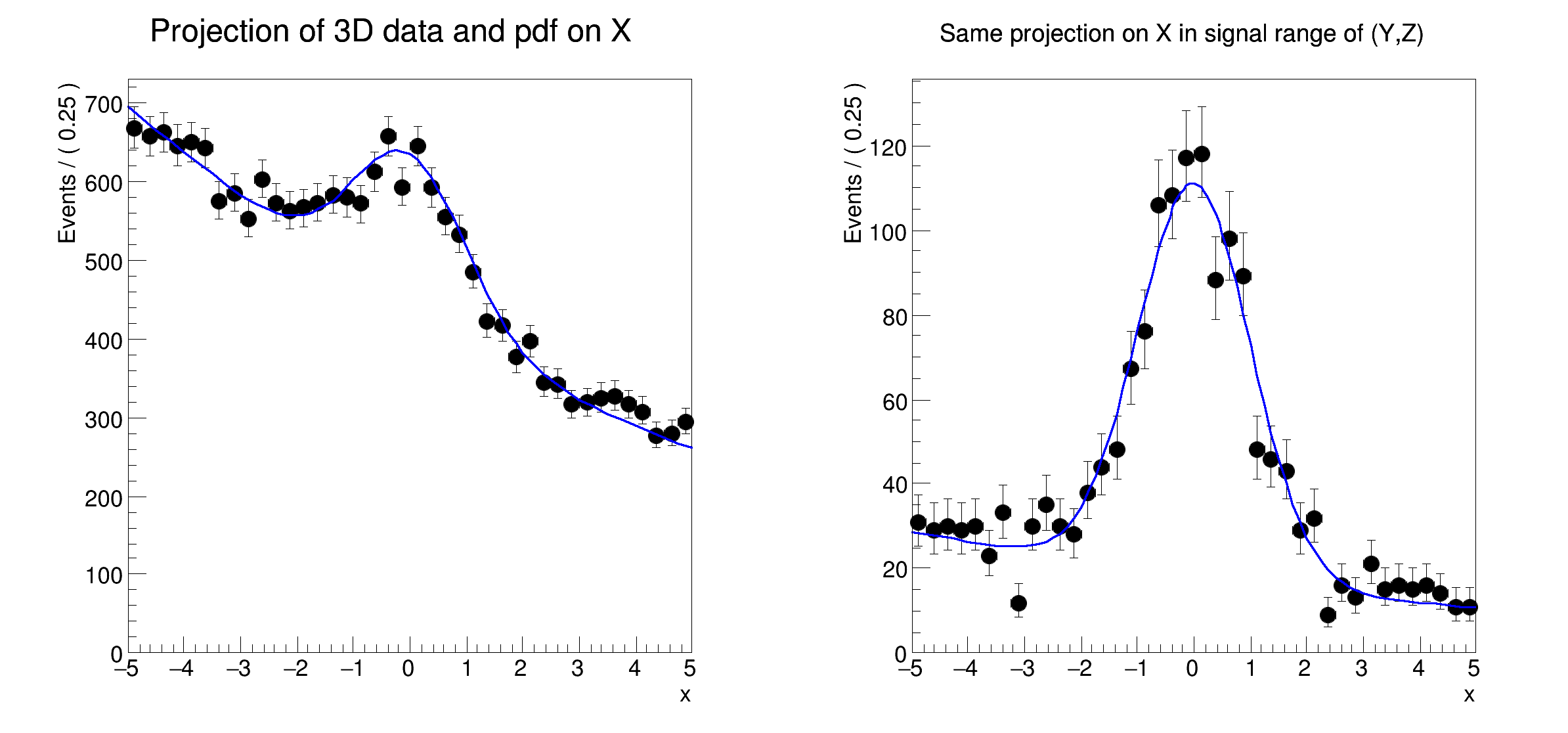ROOT   Reference Guiderf311_rangeplot.C File Reference

## Detailed Description

Multidimensional models: projecting p.d.f and data ranges in continuous observables␛[1mRooFit v3.60 -- Developed by Wouter Verkerke and David Kirkby␛[0m
Copyright (C) 2000-2013 NIKHEF, University of California & Stanford University
[#1] INFO:Plotting -- RooAbsReal::plotOn(model) plot on x integrates over variables (y,z)
[#1] INFO:Eval -- RooRealVar::setRange(y) new range named 'sigRegion' created with bounds [-1,1]
[#1] INFO:Eval -- RooRealVar::setRange(z) new range named 'sigRegion' created with bounds [-1,1]
[#1] INFO:Plotting -- RooTreeData::plotOn: plotting 1692 events out of 20000 total events
[#1] INFO:Plotting -- RooAbsReal::plotOn(model) plot on x integrates over variables (y,z) in range sigRegion
#include "RooRealVar.h"
#include "RooDataSet.h"
#include "RooGaussian.h"
#include "RooConstVar.h"
#include "RooProdPdf.h"
#include "RooPolynomial.h"
#include "TCanvas.h"
#include "TAxis.h"
#include "RooPlot.h"
using namespace RooFit;
{
// C r e a t e 3 D p d f a n d d a t a
// -------------------------------------------
// Create observables
RooRealVar x("x", "x", -5, 5);
RooRealVar y("y", "y", -5, 5);
RooRealVar z("z", "z", -5, 5);
// Create signal pdf gauss(x)*gauss(y)*gauss(z)
RooGaussian gx("gx", "gx", x, RooConst(0), RooConst(1));
RooGaussian gy("gy", "gy", y, RooConst(0), RooConst(1));
RooGaussian gz("gz", "gz", z, RooConst(0), RooConst(1));
RooProdPdf sig("sig", "sig", RooArgSet(gx, gy, gz));
// Create background pdf poly(x)*poly(y)*poly(z)
RooPolynomial px("px", "px", x, RooArgSet(RooConst(-0.1), RooConst(0.004)));
RooPolynomial py("py", "py", y, RooArgSet(RooConst(0.1), RooConst(-0.004)));
RooPolynomial pz("pz", "pz", z);
RooProdPdf bkg("bkg", "bkg", RooArgSet(px, py, pz));
// Create composite pdf sig+bkg
RooRealVar fsig("fsig", "signal fraction", 0.1, 0., 1.);
RooAddPdf model("model", "model", RooArgList(sig, bkg), fsig);
RooDataSet *data = model.generate(RooArgSet(x, y, z), 20000);
// P r o j e c t p d f a n d d a t a o n x
// -------------------------------------------------
// Make plain projection of data and pdf on x observable
RooPlot *frame = x.frame(Title("Projection of 3D data and pdf on X"), Bins(40));
data->plotOn(frame);
model.plotOn(frame);
// P r o j e c t p d f a n d d a t a o n x i n s i g n a l r a n g e
// ----------------------------------------------------------------------------------
// Define signal region in y and z observables
y.setRange("sigRegion", -1, 1);
z.setRange("sigRegion", -1, 1);
// Make plot frame
RooPlot *frame2 = x.frame(Title("Same projection on X in signal range of (Y,Z)"), Bins(40));
// Plot subset of data in which all observables are inside "sigRegion"
// For observables that do not have an explicit "sigRegion" range defined (e.g. observable)
// an implicit definition is used that is identical to the full range (i.e. [-5,5] for x)
data->plotOn(frame2, CutRange("sigRegion"));
// Project model on x, integrating projected observables (y,z) only in "sigRegion"
model.plotOn(frame2, ProjectionRange("sigRegion"));
TCanvas *c = new TCanvas("rf311_rangeplot", "rf310_rangeplot", 800, 400);
c->Divide(2);
c->cd(1);
frame->GetYaxis()->SetTitleOffset(1.4);
frame->Draw();
c->cd(2);
frame2->GetYaxis()->SetTitleOffset(1.4);
frame2->Draw();
}
#define c(i)
Definition: RSha256.hxx:101
virtual RooPlot * plotOn(RooPlot *frame, const RooCmdArg &arg1=RooCmdArg::none(), const RooCmdArg &arg2=RooCmdArg::none(), const RooCmdArg &arg3=RooCmdArg::none(), const RooCmdArg &arg4=RooCmdArg::none(), const RooCmdArg &arg5=RooCmdArg::none(), const RooCmdArg &arg6=RooCmdArg::none(), const RooCmdArg &arg7=RooCmdArg::none(), const RooCmdArg &arg8=RooCmdArg::none()) const
Calls RooPlot* plotOn(RooPlot* frame, const RooLinkedList& cmdList) const ;.
Definition: RooAbsData.cxx:546
RooAddPdf is an efficient implementation of a sum of PDFs of the form.
RooArgList is a container object that can hold multiple RooAbsArg objects.
Definition: RooArgList.h:21
RooArgSet is a container object that can hold multiple RooAbsArg objects.
Definition: RooArgSet.h:28
RooDataSet is a container class to hold unbinned data.
Definition: RooDataSet.h:33
Plain Gaussian p.d.f.
Definition: RooGaussian.h:25
A RooPlot is a plot frame and a container for graphics objects within that frame.
Definition: RooPlot.h:44
TAxis * GetYaxis() const
Definition: RooPlot.cxx:1277
virtual void Draw(Option_t *options=0)
Draw this plot and all of the elements it contains.
Definition: RooPlot.cxx:712
RooPolynomial implements a polynomial p.d.f of the form.
Definition: RooPolynomial.h:28
RooProdPdf is an efficient implementation of a product of PDFs of the form.
Definition: RooProdPdf.h:31
RooRealVar represents a variable that can be changed from the outside.
Definition: RooRealVar.h:35
virtual void SetTitleOffset(Float_t offset=1)
Set distance between the axis and the axis title.
Definition: TAttAxis.cxx:294
The Canvas class.
Definition: TCanvas.h:27
RooConstVar & RooConst(Double_t val)
RooCmdArg Bins(Int_t nbin)
RooCmdArg ProjectionRange(const char *rangeName)
RooCmdArg CutRange(const char *rangeName)
Double_t y[n]
Definition: legend1.C:17
Double_t x[n]
Definition: legend1.C:17
The namespace RooFit contains mostly switches that change the behaviour of functions of PDFs (or othe...
const char * Title
Definition: TXMLSetup.cxx:67
Date
07/2008

Definition in file rf311_rangeplot.C.